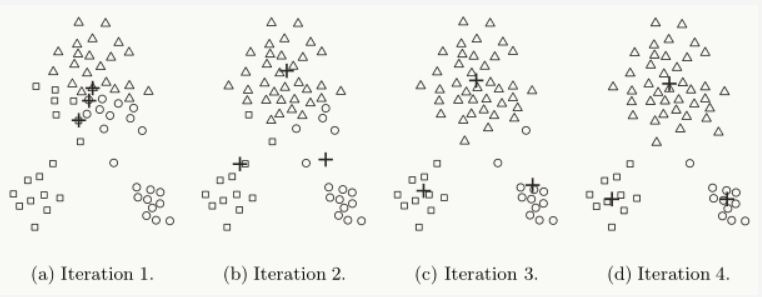# K-means 基本算法

K-means算法的核心目标是给定的数据集划分成K个簇，并给出每个数据对应的簇中心点。算法具体步骤：
（1）数据预处理,如归一化、离群点处理等
（2）随机选取K个簇中心，记为$\mu_1^{(0)},\mu_2^{(0)},…,\mu_K^{(0)}$
（3）定义代价函数：$J(c,\mu)=min_{\mu}min_{c}\sum_{i=1}^M||x_i-\mu_{c_i}||^2$
（4）令t=0,1,2,…为迭代步数，重复下面过程直到J收敛：

• 对于每个样本$x_i$，将其分配到距离最近的簇
• 对于每个类簇k,重新计算该类簇的中心

K均值算法在迭代时，假设当前J没有达到最小值，那么首先固定簇中心$\{\mu_k\}$,调整每个样例$x_i$所属的类别$c_i$来让J函数减少；然后固定$\{c_i\}$,调整簇中心$\{\mu_k\}$使J减小。这两个过程交替循环，J单调递减:当J递减到最小值时，$\{\mu_k\}$和$\{c_i\}$也同时收敛。

…..## K-means存在缺点

1. K-means是 局部最优 的，容易受到初始质心的影响;比如在下图中，因选择初始质心不恰当而造成次优的聚类结果
2. 同时K值得选取也会直接影响聚类结果，最优聚类得K值应与样本数据本身得结构信息相吻合，而这种结构信息很难去掌握，因此选取最优k值是非常困难的

## 如何选择K值

K值得选择一般基于经验和多次实验结果。可以尝试不同的K值，并将不同K值所对应的损失函数画成折线，横轴为K的取值，纵轴为误差平方和所定义的损失函数。由此可以找到一个最佳点。但是这种方法的缺点就是不够自动化。（手肘法）

Gap Statistic方法：

# K-means++算法

(1)需要人工预先确定初始K值，且该值和真实的数据分布未必吻合
(2)K均值智能收敛到局部最优，效果受到初始值很大影响
(3)易受噪声点影响
(4)样本点只能被划分到单一的类中

K-means++的思路：

# ISODATA算法

(1)预期的聚类中心个数$K_0$.在ISODATA运行过程中聚类中心可以变化，$K_0$是一个用户指定的参考值，该算法的聚类中心数目变动范围也由其决定。具体地，最终输出的聚类中心数目常见范围是从$K_0$的一半，到两倍$K_0$
(2)每个类所要求的最小样本数目$N_min$。若果分裂后会导致某个子类别所包含样本数目小于该阈值，就不会对该类别进行分裂操作
(3)最大方差Sigma。用于控制某个类别中样本的分散程度，当样本的分散程度超过这个阈值时，且分裂后满足(1),进行分裂操作
(4)每个聚类中心之间所允许最小距离$D_{min}$.如果两个类靠得非常近(即这两个类别对应聚类中心之间的距离非常小)，小于该阈值时，则对这两个类进行合并操作。

# 坐标下降法(Coordinate Descent,CD)

SGD和CD都是优化损失函数的一种方法.都沿着某个方向对参数不断迭代更新,计算量为O(mn),

SGD是沿着负梯度方向对损失函数进行参数更新（又叫最速下降法）
CD是沿着坐标轴下降方向进行参数更新,这一特点决定了两者应用场景不同.

CD进行参数更新的思想:每次只变换一个维度$x_i$,其他维度都用该参数当前值$w_{k-1}$进行计算,这样每次都得到一个该维度下的最新值$w_k$,循环迭代计算,直到$w_k$与$w_{k-1}$差别不大时停止迭代。

CD的数学依据是:当一个可微凸函数F(w),在某一坐标轴上的解$w=(w_1,w_2,…,w_n)$都达到最小值时,那么此时$w=(w_1,w_2,…,w_n)$即为F(w)的全局最小值.

-------------本文结束感谢您的阅读-------------